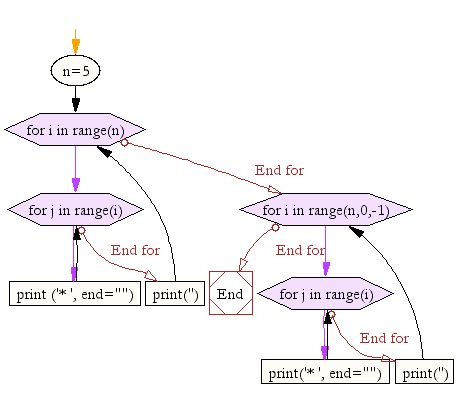﻿ Python Exercise: Construct a specified pattern, using a nested for loop - w3resource# Python Exercise: Construct a specified pattern, using a nested for loop

## Python Conditional: Exercise-4 with Solution

Write a Python program to construct the following pattern, using a nested for loop.

```* * * * * * * * * * * * * * * * * * * * * * * * *
```

Pictorial Presentation:Sample Solution:

Python Code:

``````n=5;
for i in range(n):
for j in range(i):
print ('* ', end="")
print('')

for i in range(n,0,-1):
for j in range(i):
print('* ', end="")
print('')
```
```

Sample Output:

```*
* *
* * *
* * * *
* * * * *
* * * *
* * *
* *
*
```

Flowchart:## Visualize Python code execution:

The following tool visualize what the computer is doing step-by-step as it executes the said program:

Python Code Editor:

Have another way to solve this solution? Contribute your code (and comments) through Disqus.

What is the difficulty level of this exercise?

Test your Python skills with w3resource's quiz

﻿

## Python: Tips of the Day

Returns a list with n elements removed from the beginning

Example:

```def tips_take(itr, n = 1):
return itr[:n]
print(tips_take([1, 2, 3], 5))
print(tips_take([1, 2, 3], 0))
```

Output:

```[1, 2, 3]
[]
```